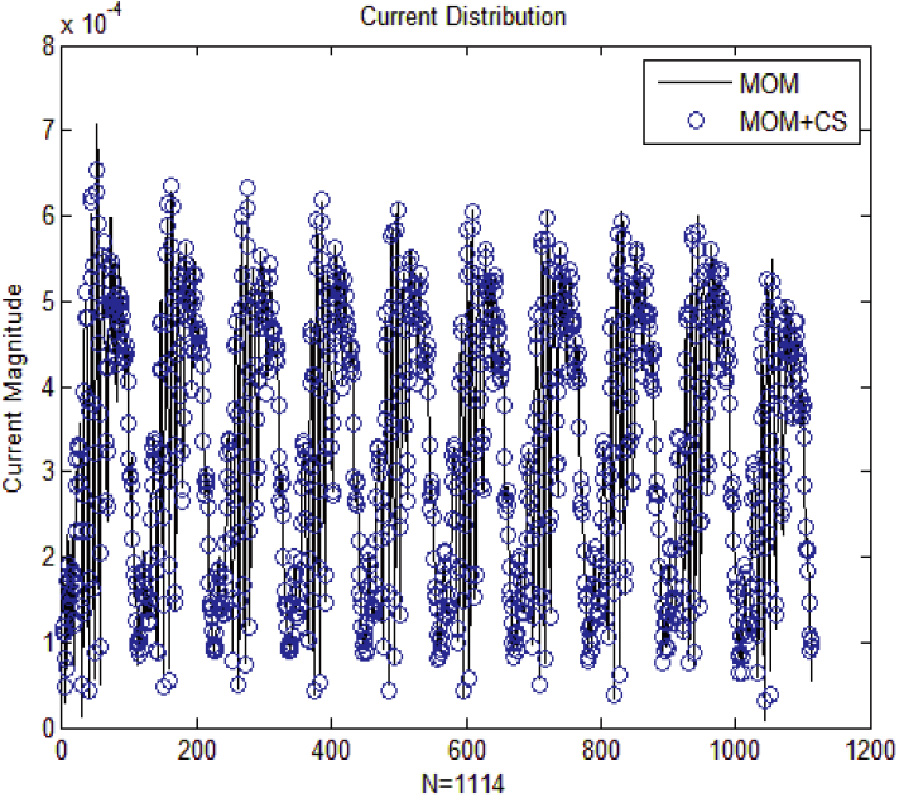# Vol. 78

Front:[PDF file] Back:[PDF file]
Latest Volume
All Volumes
All Issues
2019-02-10

#### Compressed Sensing for Fast Electromagnetic Scattering Analysis of Complex Linear Structures

By Xuehua Ma, Ming Sheng Chen, Jinhua Hu, Meng Kong, Zhi-Xiang Huang, and Xian-Liang Wu
Progress In Electromagnetics Research M, Vol. 78, 155-163, 2019
doi:10.2528/PIERM18111304

## Abstract

When method of moments (MOM) is applied to calculate electromagnetic scattering problems of the linear structures, traditional basis functions such as RWG functions are unable to satisfy the requirements of numerical discretization, so linear basis functions are constructed to discrete line structures, To avoid direct calculation of dense impedance matrix equation, compressed sensing (CS) in conjugation with appropriate transformation is introduced. Firstly, the impedance matrix equation is operated to obtain an alternative equation in transform domain. Secondly, CS is used to form an undetermined equation to be solved, under the theoretical framework of CS, and the underdetermined equation can be solved by reconstruct algorithm ​but not iterative approach. Finally, numerical simulations of single wound axial mode helical antenna and four element linear antennas array are discussed to demonstrate the efficiency and accuracy of the proposed method.

## Citation

Xuehua Ma, Ming Sheng Chen, Jinhua Hu, Meng Kong, Zhi-Xiang Huang, and Xian-Liang Wu, "Compressed Sensing for Fast Electromagnetic Scattering Analysis of Complex Linear Structures," Progress In Electromagnetics Research M, Vol. 78, 155-163, 2019.
doi:10.2528/PIERM18111304
http://www.jpier.org/PIERM/pier.php?paper=18111304

## References

1. Xu, X. W., B. Huo, and M. He, "Exact modeling for the influence of the von karman radome on antennas," Transactions of Beijing Institute of Technology, 532-535, Beijing, China, 2006.

2. Zhao, W. J. and L. W. Li, "Efficient analysis of antenna radiation in the presence of airborne dielectric radomes of arbitrary shape," IEEE Transaction on Antennas and Propagation, Vol. 53, 32-35, 2005.

3. Ma, Y., "Characteristic analysis of several wire antennas attached to an arbitrary faceted conducting body,", University of Electronic Science and Technology, Chengdu, China, 2002.

4. Harrington, R. F., Field Computation by Moment Methods, 5-90, IEEE Press, New York, NY, USA, 1993.
doi:10.1109/9780470544631

5. Rao, S. M., D. R. Wilton, and A. W. Glisson, "Electromagnetic scattering by surfaces of arbitrary shape," IEEE Transaction on Antennas and Propagation, 409-418, 1982.
doi:10.1109/TAP.1982.1142818

6. Ji, Z., T. K. Sarkar, B. H. Jung, Y. S. Chung, M. S. Palma, and M. Yuan, "A stable solution of time domain electric field integral equation for thin-wire antennas using the laguerre polynomials," IEEE Transactions on Antennas and Propagation, Vol. 52, 2641-2649, 2004.
doi:10.1109/TAP.2004.834437

7. Wagner, R. L. and W. C. Chew, "Study of wavelets for the solution of electromagnetic integral equations," IEEE Transactions on Antennas and Propagation, 802-810, 1995.
doi:10.1109/8.402199

8. Baharav, Z. and Y. Leviatan, "Impedance matrix compression (IMC) using iteratively selected wavelet basis for MFIE formulations," Microwave and Optical Technology Letters, 145-150, 1996.
doi:10.1002/(SICI)1098-2760(19960620)12:3<145::AID-MOP7>3.0.CO;2-H

9. Baharav, Z. and Y. Leviatan, "Impedance matrix compression (IMC) using iteratively selected wavelet basis," IEEE Transactions on Antennas and Propagation, 226-233, 1998.
doi:10.1109/8.660967

10. Wang, Z., "The application of compressive sensing theory in computational electromagnetics,", University of Electronic Science and Technology of China, Chengdu, China, 2015.

11. Donoho, D. L., "Compressed sensing," IEEE Trans. Inf. Theory, Vol. 52, No. 4, 1289-1306, Apr. 2006.
doi:10.1109/TIT.2006.871582

12. Candès, E., "Compressive sampling," European Mathematical Society. Proceedings of the International Congress of Mathematicians, 1433-1450, American Mathematical Society, Madrid, 2006.

13. Chen, M. S., "Compressed sensing and its application in analysis of electromagnetic scattering problems,", Post-doctoral report of the University of Science and Technology of China, 2011.

14. Cao, X. Y., M. S. Chen, and X. L.Wu, "Sparse transform matrices and its application in calculation of electromagnetic scattering problems," Chinese Physics Letters, Vol. 2, 1-4, 2013.

15. Gui, G., A. Mehbodniya, Q. Wan, and F. Adachi, "Sparse signal recovery with OMP algorithm using sensing measurement matrix," IEICE Electronics Express, Vol. 8, No. 5, 285-290, 2011.
doi:10.1587/elex.8.285

16. Kong, M., M. S. Chen, B. Wu, and X. L. Wu, "Fast and stabilized algorithm for analyzing electromagnetic scattering problems of bodies of revolution by compressive sensing," IEEE Antennas Wireless Propag. Lett., Vol. 16, 198-201, 2017.
doi:10.1109/LAWP.2016.2569605